[Introduction]  [Signal arithmetic]  [Signals and noise]   [Smoothing]   [Differentiation]  [Peak Sharpening]  [Harmonic analysis]   [Fourier convolution]  [Fourier deconvolution]  [Fourier filter]  [Wavelets]   [Peak area measurement]  [Linear Least Squares]  [Multicomponent Spectroscopy]  [Iterative Curve Fitting]  [Hyperlinear quantitative absorption spectrophotometry] [Appendix and Case Studies]  [Peak Finding and Measurement]  [iPeak]   [iSignal]  [Peak Fitters]   [iFilter]  [iPower]  [List of downloadable software]  [Interactive tools]

###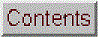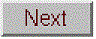Appendix V. Numerical precision of computer software (added January, 2017)

Computations carried out by computer software with non-integer numbers have a natural limit to the precision with which they can be represented; for example, the number 1/3 is represented as 0.3333333... with a large but finite number of "3"s, whereas theoretically there are an infinite string of "3"s in the decimal representation of 1/3. It's the same with irrational numbers such as "pi" and the square root of 2; they can never have a exact decimal representation. In principle, these tiny errors could accumulate in a very complex multiple-step calculation and could conceivably become a significant source of error. In the vast majority of applications to scientific computation, however, these limits will be minuscule compared to the errors and random noise that is already present in most real-world measurements. But it is best to know what those numerical limits are, under what circumstances they might occur, and how to minimize them.

Multicomponent spectroscopy. Probably the most common calculation where numerical precision is an issue is in the matrix methods that are used in multicomponent spectroscopy. In the derivation of the Classical Least Squares (CLS) method, the matrix inverse is used to solve large systems of linear equations. The matrix inverse is a standard function in programming languages such as Matlab, Octave, Wolfram's Mathematica, and in spreadsheets. But if you use that function in Matlab, the function name ("inv") is automatically flagged by the editor with the following warning:

"For solving a system of linear equations, the inverse of a matrix is primarily of theoretical value. Never use the inverse of a matrix to solve a linear system Ax=b with x=inv(A)*b, because it is slow and inaccurate.... Instead of multiplying by the inverse, use matrix right division (/) or matrix left division (\). That is: Replace inv(A)*b with A\b...[and]...replace b*inv(A) with b/A"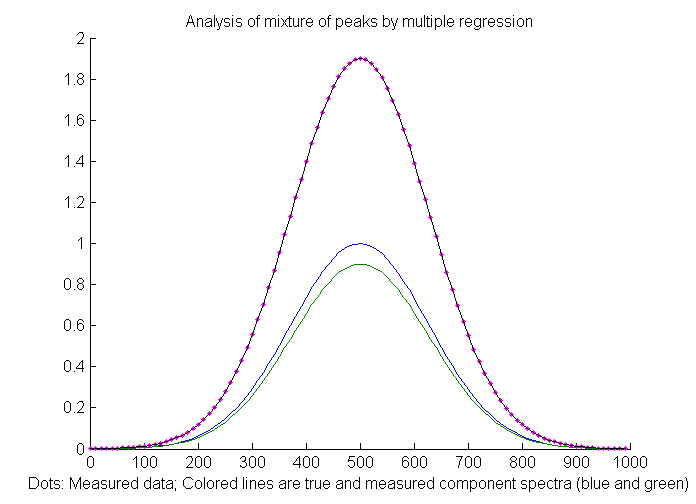"Slow and inaccurate"? OK, now I'm scared. But how serious a problem is this really in actual applications? To answer that question, the Matlab/Octave script RegressionNumericalPrecisionTest.m applies the CLS method to a mixture of two very closely-spaced noiseless overlapping Gaussian peaks (blue and green lines in the figure on the left) using three different mathematical formulations of the least-squares calculation that give different results. (The peaks must not have any added random noise, because here we are focusing on the errors caused by the computer itself and not, as in everywhere else in this essay, on external physical noise). The difficulty of such a measurement depends on the ratio of the peak separation to the peak half-width; small ratios mean very highly overlapped peaks which are hard to measure accurately. In this example the separation-to-width ratio is 0.0033, which is very small (i.e. difficult); this is equivalent to trying to measure a mixture of two absorption spectroscopy peaks that are 300 nm wide and separated by only 1 nm, a tiny difference that you wouldn't even notice with the naked eye. The results of this script shows that the matrix inverse ("inv") method does indeed have an error thousands of times larger than the method using matrix division, but even the matrix division error is still very small. Practically, the difference between these methods is unlikely to be significant when applied to real experimental data, because even the tiniest bit of signal instability (like that caused by small changes in the temperature of the sample or random noise in the signal, which you can simulate in line 15) produces a far greater error. So basically that warning message is the voice of a mathematician or computer programmer, not an experimental scientist.

Analog-to-digital resolution.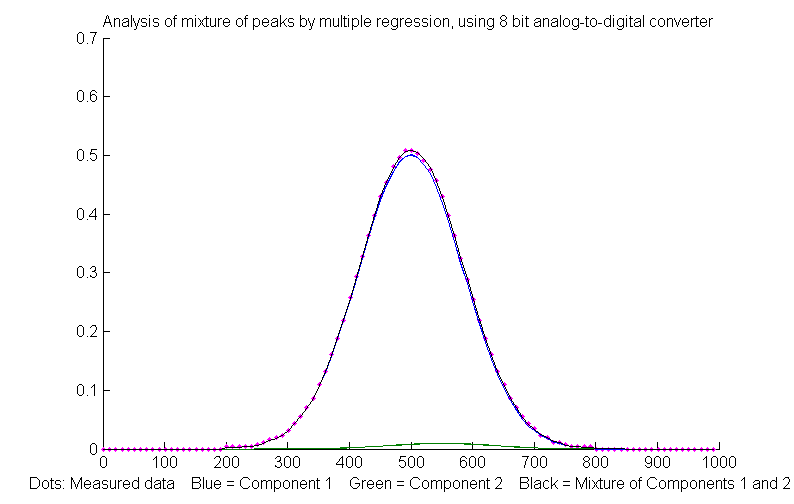Potentially more significant than the computer's numerical resolution is the resolution of the analog-to-digital converter (ADC) that is used to convert analog signals (e.g. voltage) to a number. The Matlab/Octave script RegressionADCbitsTest.m demonstrates this, with two slightly overlapping Gaussian bands with a large (50-fold) difference in peak height (blue and green lines in the figure on the right); in this case the separation to width ratio is 0.25, much larger (i.e. easier) than the previous example. For this example, the simulation shows that the relative percent errors of peak height measurement is 0.19% for the larger peak and 6.6% for the smaller peak. You can change the resolution of the simulated analog-to-digital converter in number of bits (line 9). The amplitude resolution of an  analog-to-digital converter is 2 raised to the power of the number of bits. Common ADC resolutions are 10, 12, and 14 bits, corresponding to resolutions of one part in 1024, 4096 and 16384, respectively. Of course, the effective resolution for the smaller peak in this case is 50 times less, and you can't simply turn up the amplification on the smaller peak without overloading the ADC for the larger one.

Surprisingly, if most of the noise in the signal is this kind of digitization noise, it may actually help to
add some additional random noise (specified in line 10 in this script), as was seen in appendix I.Differentiation.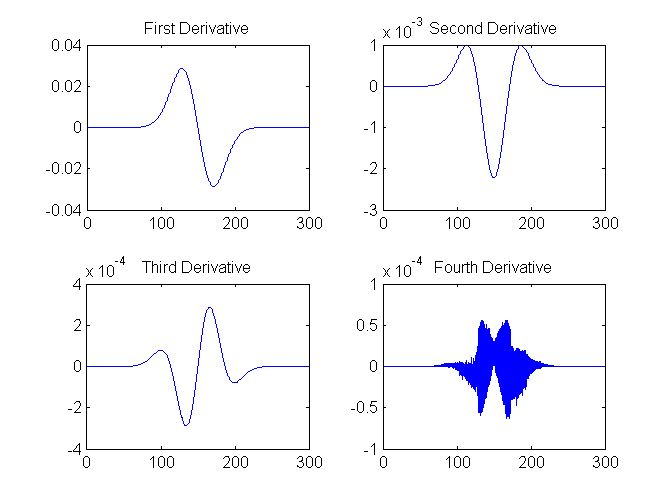Another application where you can see numerical precision noise is in differentiation, which involves the subtraction of very nearly equal adjacent numbers in a data series. The self-contained Matlab/Octave function DerivativeNumericalPrecisionDemo.m shows how the numerical precision limits of the computer effects the first through fourth derivatives of a Gaussian band that is very finely sampled (over 16,000 points in the half-width in this case) and that has no added noise. The plot on the left shows the four waveforms in Figure 1, left, and their frequency spectra in Figure 2, below. The numerical precision limits of the computer creates random noise at very high frequencies, which are emphasized by differentiation. In the frequency spectra below, the big low-frequency bump near a frequency of 10-2 is the signal and everything above that is numerical noise. The lower-order derivatives are seldom a problem, but by the time you reach the fourth derivative, those noise frequencies approach the strength of the signal frequencies, as you can see in the frequency spectrum of the fourth derivative in the lower right. But this noise is only a very high-frequency noise, so smoothing with as little as a 3-point sliding average smooth removes most of it (click to view).

The numerical errors of derivative calculations can be reduced somewhat by using the Fourier Transform method (reference 88), but the errors are spread over the entire signal period.

An alternative derivative method based on the Fourier Transform has slightly lower numerical errors but is seldom used in practice (reference 88).) The fundamental difference between the two is that the finite difference method works locally, on one small segment of the data at a time, whereas the FT method works globally, because each frequency in the Fourier representation extends throughout the entire time domain. The Matlab/Octave script FDvsFTderivative.m compares the numerical errors of finite difference (FD) and Fourier transform (FT) methods of differentiation. It creates a very broad, finely sampled Gaussian peak and then computes its fourth derivative both ways. (The noise is caused only by software numerical resolution limitations). The result is that the numerical errors are lower for the FT method near the peak but are much greater far from the peak center.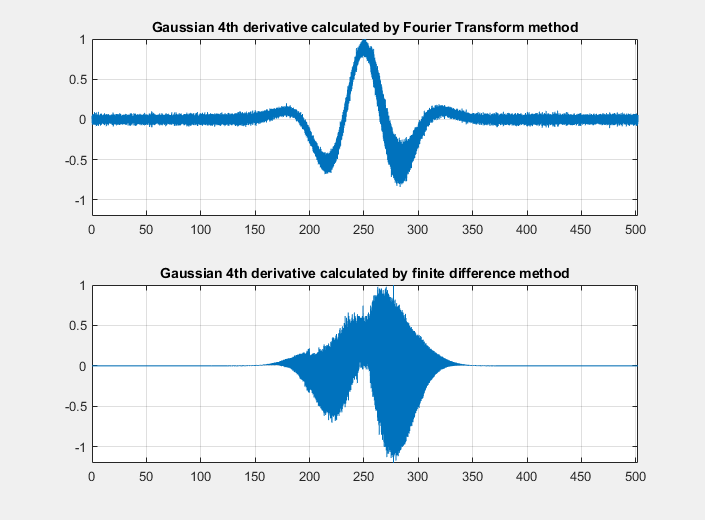Smoothing
. Finally, there might potentially be a numerical problem with the fastsmooth algorithm, covered in the section on smoothing, because it is a recursive algorithm that uses the results of a previous step in the calculation to calculate the next step. The Matlab/Octave script FastsmoothNumericalPrecisionTest.m demonstrates the numerical precision of fastsmooth.m. Even for 4000-point Gaussian smooth applied to a 100,000 point signal, the numerical noise relative standard deviation is only .00027%, and most of that occurs in the edges of the signal (first 4000 and last 4000 points); the error over 90% of the signal is orders of magnitude less. This would be a negligible problem in most cases.

This page is part of "A Pragmatic Introduction to Signal Processing", created and maintained by Prof. Tom O'Haver , Department of Chemistry and Biochemistry, The University of Maryland at College Park. Comments, suggestions and questions should be directed to Prof. O'Haver at toh@umd.edu. Updated July, 2022.